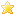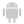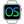Home |Sign in | EnglishHelpWLanguageWLanguage functionsStandard functionsFunctions for managing queues, stacks, lists and arraysArray functionsPrefixed syntaxAddAddColumnAddLineAddReferenceArrayAddArrayAddLineArrayAddReferenceArrayAddSortedArrayCopyArrayCountArrayDeleteArrayDeleteAllArrayDeleteDuplicateArrayDeleteLineArrayInfoArrayInsertArrayInsertLineArrayInsertReferenceArrayMoveArrayMoveLineArrayReverseArraySeekArraySeekByProcedureArrayShuffleArraySortArraySwapLineArrayToCSVArrayToStringCSVToArrayCheckAllFalseCheckAllTrueCheckAnyFalseCheckAnyTrueConstants for managing the arraysDeleteDeleteAllDeleteColumnDeleteLineFileToArrayFunctions for managing arraysInsertInsertColumnInsertLineInsertReferenceMoveMoveColumnMoveLineSeekSeekByProcedureSortStringToArraySwapLineNew WINDEV, WEBDEV, WINDEV Mobile 25 feature!
• Operating mode
• Existing computations
• Equivalence
WINDEVWEBDEVWINDEV MobileOthersReduce (Function)
In french: Reduce
Applies a computation to each element of a WLanguage array.
Example
arrValue is array of int = [1,2,3,4,5]
TheSum is integer
TheSum = Reduce(arrValue, (Value, TheSum) => { RESULT Value + TheSum }, 0)

// note: this example is for illustrative purposes ; to find the sum of elements of an array,
// it is preferable to use the Sum function
Syntax
<Result> = Reduce(<Array> , <Operation to perform> [, <Initial value>])
<Result>: Type corresponding to the result
Result of the requested reduce operation.
<Array>: WLanguage array
Name of Array variable to use. This array must be a one-dimensional array.
<Operation to perform>: WLanguage procedure
Name of WLanguage procedure to run. This procedure can be:
• a global or local procedure,
• an internal procedure.
It is also possible to directly use a lambda.
<Initial value>: Optional parameter, type corresponding to the initial value
Initial value to use the first time the <Operation to perform> procedure is run.
Remarks

Operating mode

The <Operation to perform> procedure is called a first time with two parameters:
• the first element of the array,
• the initial value specified with <Initial value>.
The procedure is then called for all other elements of the array with two parameters:
• the element of the array,
• the value returned by the previous call.
Illustrative example
Let's study the following example:
arrValue is array of int = [1,2,3,4,5]
TheSum is integer
TheSum = Reduce(arrValue, (Value, TheSum) => { RESULT Value + TheSum }, 0)
In this example, the code:
TheSum = Reduce(arrValue, (Value, TheSum) => { RESULT Value + TheSum }, 0)
is equivalent to the following code:

RESULT Value + Total
END
With this internal procedure, the calculation can be broken down as follows:
• IntermediateResult1 = Add (t, InitialValue): in the example Add( 1, 0 ) -> 1
• IntermediateResult2 = Add (t, IntermediateResult1): in the example Add( 2, 1 ) -> 3
• ...
Remark: this example is for illustrative purposes ; to find the sum of elements of an array, it is preferable to use Sum.

Existing computations

The most common computations are available in WLanguage:Versions 17 and laterMeanNew in version 17MeanMean Calculates the mean of several elements: elements found in an array, numeric values, ...Versions 17 and laterSumNew in version 17SumSum Calculates the sum of the array elements.

Equivalence

The FOR EACH syntax also allows you to browse through the elements of an array to perform reduce operations.
Business / UI classification : Neutral code
Component : wd250vm.dll
Minimum version required
• Version 25